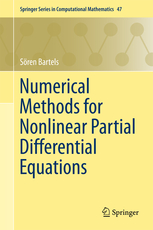# BücherS. Bartels Numerical Approximation of Partial Differential Equations Springer Texts in Applied Mathematics, Vol. 64, 2016

Finite element methods for approximating partial differential equations have reached a high degree of maturity, and are an indispensible tool in science and technology. This textbook aims at providing a thorough introduction to the construction, analysis, and implementation of finite element methods for model problems arising in continuum mechanics. The first part of the book discusses elementary properties of linear partial differential equations along with their basic numerical approximation, the functional-analytical framework for rigorously establishing existence of solutions, and the construction and analysis of basic finite element methods. The second part is devoted to the optimal adaptive approximation of singularities and the fast iterative solution of linear systems of equations arising from finite element discretizations. In the third part, the mathematical framework for analyzing and discretizing saddle-point problems is formulated, corresponding finte element methods are analyzed, and particular applications including incompressible elasticity, thin elastic objects, electromagnetism, and fluid mechanics are addressed. The book includes theoretical problems and practical projects for all chapters, and an introduction to the implementation of finite element methods.S. Bartels Numerik 3x9 Springer-Lehrbuch, 2016

Dieses Buch bietet eine Einführung in Methoden zur praktischen Lösung mathematischer Probleme, wie der Bestimmung von Eigenwerten, der Approximation und Integration von Funktionen und der näherungsweisen Lösung gewöhnlicher Differenzialgleichungen. Vorausgesetzt werden nur Grundkenntnisse aus der linearen Algebra und Analysis sowie elementare Programmiererfahrungen. Lernziele, Tests zur Selbstüberprüfung und Anwendungsaufgaben am Ende jedes Kapitels vertiefen das Verständnis. Im Anhang des Buchs finden sich unter anderem eine umfangreiche Aufgabensammlung, detaillierte Beschreibungen für Programmierprojekte, Einführungen in die Programmiersprachen Matlab und C und einige Beispielprogramme.S. Bartels Numerical Methods for Nonlinear Partial Differential Equations Springer Series in Computational Mathematics, Vol. 47, 2015

The description of many interesting phenomena in science and engineering leads to infinite-dimensional minimization or evolution problems that define nonlinear partial differential equations. While the development and analysis of numerical methods for linear partial differential equations is nearly complete, only few results are available in the case of nonlinear equations. This monograph devises numerical methods for nonlinear model problems arising in the mathematical description of phase transitions, large bending problems, image processing, and inelastic material behavior. For each of these problems the underlying mathematical model is discussed, the essential analytical properties are explained, and the proposed numerical method is rigorously analyzed. The practicality of the algorithms is illustrated by means of short implementations.